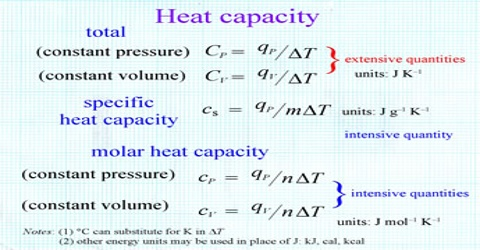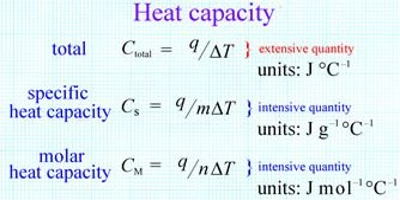# Heat Capacity and Molar Heat Capacity

Molar heat capacity is calculated of the amount of heat needed to raise the temperature of one mole of a pure substance by one degree K. The molar heat capacity is a more useful quantity to work with in chemistry. It is the energy required to raise one mole of any substance by one degree Celsius. It is a common experience that the temperature of a substance (a system) increases when heat is supplied to it. The resulting temperature increase, however, depends on the ‘heat capacity’ of the substance. The heat capacity C is defined as;

C = q/∆T … … …. (1)

where, q is the amount of heat absorbed by the system and ∆T (=Tf —Ti) is the temperature change. The heat capacity, C, is defined as, “the amount of heat energy required to raise the temperature of a system by one degree”. If the temperature is expressed in Kelvin and q is in joule. C is expressed as J K-1; or in J per degree Celsius, if the temperature is in degree Celsius. Calculation of the amount of heat energy absorbed or released by a system is a simple operation by rearranging equation (1). Thus,

q = C x ∆T … …. ….. (2)C is an extensive property since it is dependent on the amount of substance present. It is, however, convenient to express the heat capacity as an intensive property when it is independent of the amount of substance present. Two other terms, specific heat capacity (CS), or molar heat capacity (Cm) are used for this purpose. By definition,

CS = C/m = JK-1/g = JK-1g-1

and Cm = C/n = JK-1/mol = JK-1mol-1

Here, m is the mass in g and n is the number of moles of the substance of which the heat capacity is C. Now heat energy can be supplied to the system at constant volume as well as at constant pressure. Accordingly the heat capacities are distinguished as CV specific heat at constant volume, or CP specific heat at constant pressure.